# Arkady Tsurkov – IBN-varieties of algebras

Federal University Of Rio Grande Do Norte, Brazil
We consider in a class of all universal algebras of a signature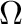some variety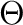. We denote by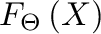the free algebra of this variety generated by the set of free generators. We consider only finitely generated free algebras. We denote by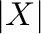the quantity of the elements of the set.

Definition 1   We say that the varietyis an IBN-variety (or variety which has an IBN propriety), if fromwe can conclude that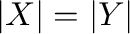.

The concept of variety with IBN (invariant basic number) propriety first appeared in ring theory. It is known that if we consider some field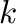, the vector spaceover this field such that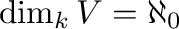ring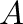of all linear operators on this vector space (), then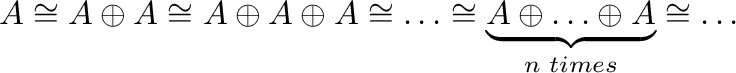, i.e., the variety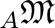of all rights modules over the ringhas not the IBN propriety.

The proving of the IBN propriety of some variety is very important in universal algebraic geometry. This is a milestone in the study of the relation between geometric and automorphic equivalences of algebras of this variety.

We will look at some examples where the IBN property of certain varieties can be proved directly. For example for every signaturethe variety defined by the empty set of identities has the IBN property.

We will discuss very simple but very useful

Theorem 1   Ifsome varieties of universal algebras of a signature,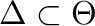andis an IBN-variety thenis also an IBN-variety.

We will consider applications of this theorem.

We will consider many-sorted universal algebras as well as one-sorted. So all concepts and all results will by generalized for the many-sorted case.